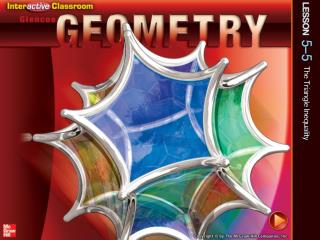DownloadDownload PresentationSplash Screen

# Splash Screen

Download Presentation## Splash Screen

- - - - - - - - - - - - - - - - - - - - - - - - - - - E N D - - - - - - - - - - - - - - - - - - - - - - - - - - -
##### Presentation Transcript

1. Splash Screen

2. Five-Minute Check (over Lesson 5–4) CCSS Then/Now Theorem 5.11: Triangle Inequality Theorem Example 1: Identify Possible Triangles Given Side Lengths Example 2: Standardized Test Example: Find Possible Side Lengths Example 3: Real-World Example: Proof Using Triangle Inequality Theorem Lesson Menu

3. A. ABC is a right triangle. B. A = D C.AB = DE D.ΔABC / ΔDEF ___ ___  State the assumption you would make to start an indirect proof of the statement.ΔABC ΔDEF 5-Minute Check 1

4. State the assumption you would make to start an indirect proof of the statement.RS is an angle bisector. ___ ___ A.RS is a perpendicular bisector. B.RS is not an angle bisector. C.R is the midpoint of ST. D.mR = 90° ___ ___ 5-Minute Check 2

5. State the assumption you would make to start an indirect proof of the statement.X is a right angle. A.X is a not a right angle. B.mX < 90° C.mX > 90° D.mX = 90° 5-Minute Check 3

6. State the assumption you would make to start an indirect proof of the statement.If 4x – 3 ≤ 9, then x ≤ 3. A.4x – 3 ≤ 9 B.x > 3 C.x > 1 D.4x ≤ 6 5-Minute Check 4

7. State the assumption you would make to start an indirect proof of the statement.ΔMNO is an equilateral triangle. A.ΔMNO is a right triangle. B.ΔMNO is an isosceles triangle. C.ΔMNO is not an equilateral triangle. D.MN = NO = MO 5-Minute Check 5

8. Which statement is a contradiction to the statement that AB CD? ___ ___ A.AB = CD B.AB > CD C.CD  AB D.AB ≤CD ___ ___ 5-Minute Check 6

9. Content Standards G.CO.10 Prove theorems about triangles. G.MG.3 Apply geometric methods to solve problems (e.g., designing an object or structure to satisfy physical constraints or minimize cost; working with typographic grid systems based on ratios). Mathematical Practices 1 Make sense of problems and persevere in solving them. 2 Reason abstractly and quantitatively. CCSS

10. You recognized and applied properties of inequalities to the relationships between the angles and sides of a triangle. • Use the Triangle Inequality Theorem to identify possible triangles. • Prove triangle relationships using the Triangle Inequality Theorem. Then/Now

11. Concept

12. A. Is it possible to form a triangle with side lengths of 6 , 6 , and 14 ? If not, explain why not. 1 1 1 __ __ __ 2 2 2 Identify Possible Triangles Given Side Lengths Example 1

13. Identify Possible Triangles Given Side Lengths B. Is it possible to form a triangle with side lengths of 6.8, 7.2, 5.1? If not, explain why not. Example 1

14. A. yes B. no Example 1

15. B. Is it possible to form a triangle given the side lengths 4.8, 12.2, and 15.1? A. yes B. no Example 1

16. Find Possible Side Lengths In ΔPQR, PQ = 7.2 and QR = 5.2. Which measure cannot be PR? A 7 B 9 C 11 D 13 Example 2

17. In ΔXYZ, XY = 6, and YZ = 9. Which measure cannot be XZ? A. 4 B. 9 C. 12 D. 16 Example 2

18. Proof Using Triangle Inequality Theorem TRAVEL The towns of Jefferson, Kingston, and Newbury are shown in the map below. Prove that driving first from Jefferson to Kingston and then Kingston to Newbury is a greater distance than driving from Jefferson to Newbury. Example 3

19. Jacinda is trying to run errands around town. She thinks it is a longer trip to drive to the cleaners and then to the grocery store, than to the grocery store alone. Determine whether Jacinda is right or wrong. A. Jacinda is correct, HC + CG > HG. B. Jacinda is not correct, HC + CG < HG. Example 3